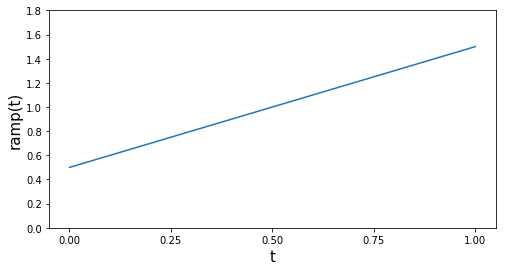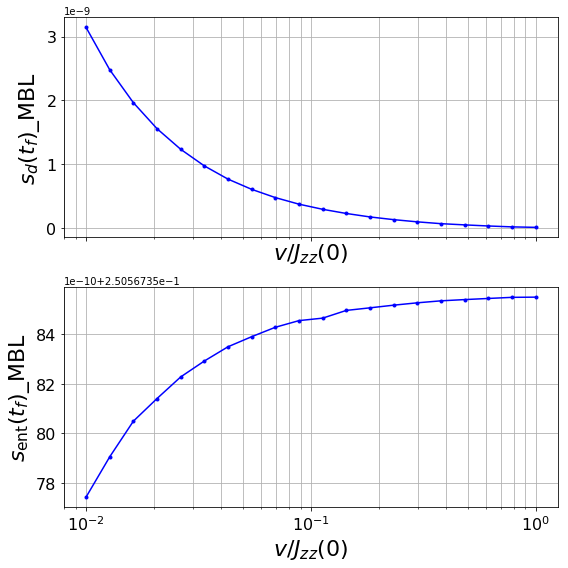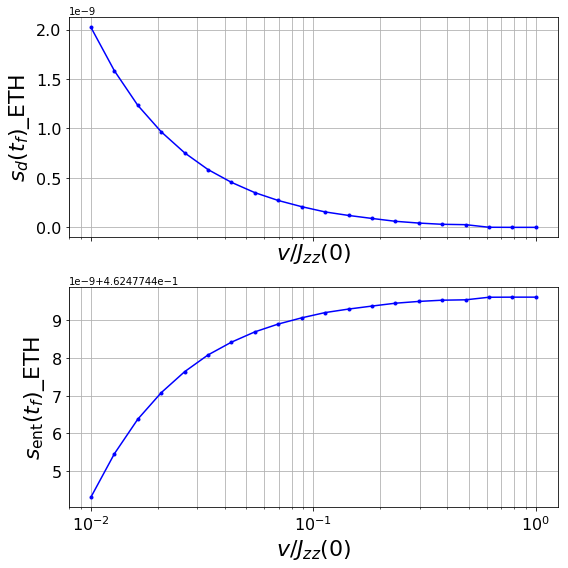# Many-Body Localization¶

## Adiabatic Control of Parameters¶### Notebook by Marco Tavora¶Fig.1: Occupation discontinuity as a function of disorder strength and energy density for spinless fermions in 1D with nearest-neighbor repulsion and diagonal disorder (Source: Bera S., Schomerus H., Heidrich-Meisner F., and H. Bardarson J..

## Introduction¶

[go back to the top]

Let us consider a closed quantum system:The (time-dependent) Hamiltonian, known to exhibit a MBL dynamical phase transition reads:

\begin{eqnarray} H(t) = \sum\limits_{i = 0}^{L - 2} {\left[ {\frac{{{J_{xy}}}}{2}(S_{i + 1}^ + S_i^ - + S_i^ + S_{i + 1}^ - ) + {J_{zz}}(t)S_{i + 1}^zS_i^z} \right]} + \sum\limits_{i = 0}^{L - 1} {{h_i}S_i^z} ,\,\,\,\,\,\,\,\,\,\,{J_{zz}}(t) = (1/2 + vt). \end{eqnarray}

The parameters here are:

• $h_j$: random field in the $z$-direction, uniformly distributed within the interval $[-h_0,h_0]$
• $J_{zz}(t)$ is modulated adiabatically and with ramp speed $v$

## Can the system adiabatically follow the ramp?¶

[go back to the top]

It has been argued quite recently that the adiabatic theorem cannot be applied to disordered systems.

We will address here the following question: can the system follow the ramp (varying $v$) adiabatically, a least in part? More concretely, we will evolve the system from $t_i=0$ to $t=(2v)^{-1}$.

To assure tipicality, the initial state we will chose will be an eigenstate of the initial Hamiltonian with the energy closest to the middle spectrum.

## Indicators¶

[go back to the top]

The following indicators will be used to verify if the system can follow the ramp adiabatically:

• diagonal entropy density
• entanglement entropy density

We will calculate the (disorder-averaged) expressions for bioth entropies in terms of the velocity parameter.

### Diagonal entropy density¶

The diagonal entropy density reads:

$${s_d}(t) = - \frac{1}{L}\,{\rm{Tr}}\,{\rho _d}(t)\ln {\rho _d}(t),\,\,\,\,\,\,\,\,\,{\rho _d}(t) = \sum\nolimits_n {{{\left| {\left\langle {n|\psi (t)} \right\rangle } \right|}^2}\left| n \right\rangle \langle n|} {\rm{ }}$$

${s_d}(t)$ measures of degree of delocalization of the final state among the eigenstates $H(t)$ (e.g. if the final state of the system is a single eigenstate of $H(t)$, ${s_d}(t)=0$)

### Entanglement entropy density¶

$${s_e}(t) = - \frac{1}{{|A|}}{\rm{Tr}}{\,_A}{\rho _A}(t)\ln {\rho _A}(t),\,\,\,\,\,\,\,\,\,{\rho _A}(t) = {\rm{T}}{{\rm{r}}_{{A^c}}}\,\left| {\psi (t)} \right\rangle \langle \psi (t)|.$$

Here:

• $t$ is the final time
• $A$ is a subsystem containing the left half of the system
• $\rho_A$ is its reduced density matrix
• $|A|=1/2$.

## Code¶

[go back to the top]

### Importing packages¶

This notebook will follow very closely the documentation found here. The first step is to import the necessary Python modules. Besides some of the usual Python packages, we will need the following packages from QuSpin:

• The basis module quspin.basis to build the Hilbert space
• The operators module quspin.operators to build the Hamiltonian and operators
• quspin.tools.measurements for the entropies

### Python packages¶

The module auxiliar_functions contains auxiliar functions I wrote to make the code briefer.

In :
import sys,os
import numpy as np
import numpy as np
from time import time
import auxiliar_functions as aux
import pandas as pd
import matplotlib.pyplot as plt
%matplotlib inline


### QuSpin packages¶

In :
from quspin.operators import hamiltonian
from quspin.basis import spin_basis_1d
from quspin.tools.measurements import ent_entropy, diag_ensemble


## Parameters¶

[go back to the top]

We need to choose the following parameters:

• The number of disorder realizations n_real
• The number of processes used for parallelisation n_jobs
• System size L
• Jxy interaction
• Jzz_0 interaction
• MBL disorder strength h_MBL
• Delocalized disorder strength h_ETH
In :
n_real, n_jobs =20, 2
L, Jxy, Jzz_0, h_MBL, h_ETH =10, 1.0, 1.0, 3.9, 0.1


We create an array vs for the velocity $v$:

In :
vs = np.logspace(-2.0,0.0,num=20,base=10)
type(vs)

Out:
numpy.ndarray
In :
print('The ramp speeds are:')
pd.DataFrame(np.array(list(vs)).reshape(vs.shape,1), columns = list("v")).T

The ramp speeds are:

Out:
0 1 2 3 4 5 6 7 8 9 10 11 12 13 14 15 16 17 18 19
v 0.01 0.012743 0.016238 0.020691 0.026367 0.033598 0.042813 0.054556 0.069519 0.088587 0.112884 0.143845 0.183298 0.233572 0.297635 0.379269 0.483293 0.615848 0.78476 1.0

## Building the Hamiltonian¶

[go back to the top]

We first need to build the ramp function ramp(t):

### ramp function¶

In :
v = 1.0

def ramp(t):
return (0.5 + v*t)

ramp_args=[]

In :
fig = plt.figure(figsize=(8, 4));
ramp_list = [ramp(t)
for t in range(2)]
plt.plot(ramp_list)
plt.xlabel('t', fontsize=15)
plt.ylabel('ramp(t)',
fontsize=15)
plt.yticks(np.arange(0, 2, step=0.2))
plt.xticks(np.arange(0, 1.1, step=0.25))
plt.show();Following the syntax described in the appendix, the lines of code needed to build the XXZ Hamiltonian are (we will compute the basis in the 0-total magnetisation sector):

In :
basis = spin_basis_1d(L,Nup=L//2, pauli=False)

J_zz, J_xy = [[Jzz_0,i,i+1]
for i in range(L-1)], [[Jxy/2.0,i,i+1]
for i in range(L-1)]

static, dynamic = [["+-",J_xy],["-+",J_xy]], [["zz",J_zz,ramp,ramp_args]]

In :
H_XXZ = hamiltonian(static,dynamic,basis=basis,dtype=np.float64)

Hermiticity check passed!
Symmetry checks passed!
Particle conservation check passed!


The function realization, shown a few cells below, returns two numpy arrays, one for each phase (MBL and ETH). Inside each array we put the value of both entropies corresponding to each $v$ (as columns).

We need to produce many disorder realisations and average them. The function realization computes $s_d$ and $s_e$ for one realization.

The following comments are relevant:

• ti is the initial time
• v is chosen to be a global variable
• unscaled_fields is a random field inside [-1.0,1.0] for each lattice site
In :
unscaled_fields = -1+2*np.random.ranf((basis.L,))
print('random field:')
pd.DataFrame(np.array(list(unscaled_fields)).reshape(unscaled_fields.shape,
1), columns = ['random field']).T

random field:

Out:
0 1 2 3 4 5 6 7 8 9
random field 0.177147 0.837917 0.527189 -0.861374 -0.279513 0.720321 -0.947146 -0.572016 0.686134 -0.619889
• h_z is a $z$-field operator site-coupling list
In :
def realization(vs, H_XXZ, basis, real):

ti = time()
global v
np.random.seed()

unscaled_fields=-1+2*np.random.ranf((basis.L,))

h_z=[[unscaled_fields[i],i] for
i in range(basis.L)]
disorder_field = [["z", h_z]]

no_checks={"check_herm":False,
"check_pcon":False,
"check_symm":False}

Hz=hamiltonian(disorder_field,[],
basis=basis,
dtype=np.float64,
**no_checks)

H_MBL, H_ETH = H_XXZ + h_MBL*Hz, H_XXZ + h_ETH*Hz

v=1.0

eigsh_args={"k":2,"which":"BE",
"maxiter":1E4,
"return_eigenvectors":False}

Emin,Emax=H_MBL.eigsh(time=0.0,**eigsh_args)
E_inf_temp=(Emax+Emin)/2.0

E,psi_0=H_MBL.eigsh(time=0.0,k=1,
sigma=E_inf_temp,maxiter=1E4)
psi_0=psi_0.reshape((-1,))

E_final,V_final=H_MBL.eigh(time=(0.5/vs[-1]))

def _do_ramp(psi_0,H,basis,v,E_final,V_final):

t_f = 0.5/v
psi = H.evolve(psi_0,0.0,t_f)
subsys = range(basis.L//2)
Sent = ent_entropy(psi,basis,
chain_subsys=subsys)["Sent"]
S_d = diag_ensemble(basis.L,psi,
E_final,
V_final,
Sd_Renyi=True)["Sd_pure"]

return np.asarray([S_d,Sent])

run_MBL=[_do_ramp(psi_0,H_MBL,basis,v,E_final,V_final)
for v in vs]

run_MBL=np.vstack(run_MBL).T

v=1.0

Emin,Emax=H_ETH.eigsh(time=0.0,**eigsh_args)
E_inf_temp=(Emax+Emin)/2.0

E,psi_0=H_ETH.eigsh(time=0.0,k=1,
sigma=E_inf_temp,maxiter=1E4)
psi_0=psi_0.reshape((-1,))

E_final,V_final=H_ETH.eigh(time=(0.5/vs[-1]))

run_ETH=[_do_ramp(psi_0,
H_ETH,basis,
v,E_final,
V_final) for v in vs]
run_ETH=np.vstack(run_ETH).T

return run_MBL,run_ETH


For the first realization:

In :
print('velocity grid:\n')
print([round(el,3)
for el in list(vs)])

velocity grid:

[0.01, 0.013, 0.016, 0.021, 0.026, 0.034, 0.043, 0.055, 0.07, 0.089, 0.113, 0.144, 0.183, 0.234, 0.298, 0.379, 0.483, 0.616, 0.785, 1.0]

In :
s_d_mbl = list(realization(vs,H_XXZ,basis,1))
s_e_mbl = list(realization(vs,H_XXZ,basis,1))
s_d_eth = list(realization(vs,H_XXZ,basis,1))
s_e_eth = list(realization(vs,H_XXZ,basis,1))

In :
results = pd.DataFrame({'s_d of MBL': s_d_mbl,
's_e of MBL': s_e_mbl,
's_d of ETH': s_d_eth,
's_e of ETH': s_e_eth})

results.index.name = 'realization'

In :
results.head()

Out:
s_d of MBL s_e of MBL s_d of ETH s_e of ETH
realization
0 1.000856e-13 0.28621 1.932562e-09 0.464687
1 1.000634e-13 0.28621 1.494026e-09 0.464687
2 1.000412e-13 0.28621 1.170946e-09 0.464687
3 1.000856e-13 0.28621 9.089409e-10 0.464687
4 1.001078e-13 0.28621 7.054291e-10 0.464687

### n_real disorder realisations¶

In :
if __name__ == '__main__':

data = np.asarray([realization(vs,H_XXZ,basis,i)
for i in range(n_real)])
run_MBL,run_ETH = zip(*data)
mean_MBL = np.mean(run_MBL,axis=0)
mean_ETH = np.mean(run_ETH,axis=0)


## Plot results¶

[go back to the top]

### MBL and ETH plots¶

• subplot 1: diagonal entropy versus ramp speed
• subplot 2: entanglement entropy versus ramp speed
In :
from pylab import rcParams

rcParams['figure.figsize'] = 8, 8;

for phase in ['MBL', 'ETH']:

fig, pltarr1 = plt.subplots(2,sharex=True);
pltarr1.plot(vs,
eval('mean_{}'.format(phase)),
label = phase,
marker=".",
color="blue");
pltarr1.set_ylabel("$s_d(t_f)$" + '_' + phase,
fontsize=22);
pltarr1.set_xlabel("$v/J_{zz}(0)$",
fontsize=22);
pltarr1.set_xscale("log");
pltarr1.grid(True,which='both');
pltarr1.tick_params(labelsize=16);
pltarr1.plot(vs,
eval('mean_{}'.format(phase)),
marker=".",
color="blue");
pltarr1.set_ylabel("$s_\mathrm{ent}(t_f)$" + '_'+ phase,
fontsize=22);
pltarr1.set_xlabel("$v/J_{zz}(0)$",
fontsize=22);
pltarr1.set_xscale("log");
pltarr1.grid(True,which='both') ;
pltarr1.tick_params(labelsize=16);
plt.tight_layout();
plt.show();

Out:
[<matplotlib.lines.Line2D at 0x1c1f605400>]
Out:
Text(0,0.5,'$s_d(t_f)$_MBL')
Out:
Text(0.5,0,'$v/J_{zz}(0)$')
Out:
[<matplotlib.lines.Line2D at 0x1c1f335c18>]
Out:
Text(0,0.5,'$s_\\mathrm{ent}(t_f)$_MBL')
Out:
Text(0.5,0,'$v/J_{zz}(0)$')Out:
[<matplotlib.lines.Line2D at 0x1c1eccf080>]
Out:
Text(0,0.5,'$s_d(t_f)$_ETH')
Out:
Text(0.5,0,'$v/J_{zz}(0)$')
Out:
[<matplotlib.lines.Line2D at 0x1c1ea778d0>]
Out:
Text(0,0.5,'$s_\\mathrm{ent}(t_f)$_ETH')
Out:
Text(0.5,0,'$v/J_{zz}(0)$')## Acknowledgements¶

[go back to the top]

This notebook is heavily based on the excellent tutorial about the QuSPin package created by Phillip Weinberg and Marin Bukov.

## Appendix¶

[go back to the top]

### Building a basis¶

A few observations:

• The optional argument pauli = False prevents the code to use the Pauli matrices instead of spin matrices as needed.
• We choose half-filling and the number of spins-up is rounded down.
• Furthermore, since this Hamiltonian conserves parity (which in this case corresponds to reflection with respect to the middle of the chain), the Hilbert space is divided into two subspaces and we must choose one of them. To choose positive parity we set the optional argument pblock=1.
In :
basis = spin_basis_1d(L, pauli=False, Nup = L//2, pblock = 1)
print(' The basis vectors are given by:\n\n',basis)

 The basis vectors are given by:

reference states:
0.  |1 1 1 1 1 0 0 0 0 0>
1.  |1 1 1 1 0 1 0 0 0 0>
2.  |1 1 1 1 0 0 1 0 0 0>
3.  |1 1 1 1 0 0 0 1 0 0>
4.  |1 1 1 1 0 0 0 0 1 0>
5.  |1 1 1 1 0 0 0 0 0 1>
6.  |1 1 1 0 1 1 0 0 0 0>
7.  |1 1 1 0 1 0 1 0 0 0>
8.  |1 1 1 0 1 0 0 1 0 0>
9.  |1 1 1 0 1 0 0 0 1 0>
10.  |1 1 1 0 1 0 0 0 0 1>
11.  |1 1 1 0 0 1 1 0 0 0>
12.  |1 1 1 0 0 1 0 1 0 0>
13.  |1 1 1 0 0 1 0 0 1 0>
14.  |1 1 1 0 0 1 0 0 0 1>
15.  |1 1 1 0 0 0 1 1 0 0>
16.  |1 1 1 0 0 0 1 0 1 0>
17.  |1 1 1 0 0 0 1 0 0 1>
18.  |1 1 1 0 0 0 0 1 1 0>
19.  |1 1 1 0 0 0 0 1 0 1>
20.  |1 1 1 0 0 0 0 0 1 1>
21.  |1 1 0 1 1 1 0 0 0 0>
22.  |1 1 0 1 1 0 1 0 0 0>
23.  |1 1 0 1 1 0 0 1 0 0>
24.  |1 1 0 1 1 0 0 0 1 0>
:
101.  |0 1 1 1 1 0 0 0 1 0>
102.  |0 1 1 1 0 1 1 0 0 0>
103.  |0 1 1 1 0 1 0 1 0 0>
104.  |0 1 1 1 0 1 0 0 1 0>
105.  |0 1 1 1 0 0 1 1 0 0>
106.  |0 1 1 1 0 0 1 0 1 0>
107.  |0 1 1 1 0 0 0 1 1 0>
108.  |0 1 1 0 1 1 1 0 0 0>
109.  |0 1 1 0 1 1 0 1 0 0>
110.  |0 1 1 0 1 1 0 0 1 0>
111.  |0 1 1 0 1 0 1 1 0 0>
112.  |0 1 1 0 1 0 1 0 1 0>
113.  |0 1 1 0 1 0 0 1 1 0>
114.  |0 1 1 0 0 1 1 1 0 0>
115.  |0 1 1 0 0 1 1 0 1 0>
116.  |0 1 0 1 1 1 1 0 0 0>
117.  |0 1 0 1 1 1 0 1 0 0>
118.  |0 1 0 1 1 1 0 0 1 0>
119.  |0 1 0 1 1 0 1 1 0 0>
120.  |0 1 0 1 1 0 1 0 1 0>
121.  |0 1 0 1 0 1 1 1 0 0>
122.  |0 1 0 0 1 1 1 1 0 0>
123.  |0 0 1 1 1 1 1 0 0 0>
124.  |0 0 1 1 1 1 0 1 0 0>
125.  |0 0 1 1 1 0 1 1 0 0>
The states printed do NOT correspond to the physical states: see review arXiv:1101.3281 for more details about reference states for symmetry-reduced blocks.



### Operators¶

We will choose open boundary conditions in this case. To define the operators we use list comprehensions.

In [ ]:
def spin_operators(coupling, L):
return [[coupling,i,i+1] for i in range(L-1)]

In :
L, Jxy, Jzz, hz = 10, 1.0, 1.0, 1.0

In :
J_zz = spin_operators(Jzz, L)
J_xy = spin_operators(Jxy/2.0, L)
h_z=[[hz,i] for i in range(L)]


### Hamiltonian¶

In this case we have a static Hamiltonian. The corresponding syntax is:

In :
static, dynamic = [["+-",J_xy],["-+",J_xy],["zz",J_zz],["z",h_z]], []
H_XXZ = hamiltonian(static,dynamic,basis=basis,dtype=np.float64)

Hermiticity check passed!
Symmetry checks passed!
Particle conservation check passed!


The eigenvalues are obtained using:

In :
E = H_XXZ.eigvalsh()

print('Some eigenvalues are:\n')
print('Lowest:',[round(el,1) for el in sorted(E)[0:5]],'\n')
print('Highest:',[round(el,1) for el in sorted(E)[len(E)-5:]])

Some eigenvalues are:

Lowest: [-3.9, -3.2, -3.2, -3.0, -2.7]

Highest: [2.0, 2.0, 2.1, 2.1, 2.3]